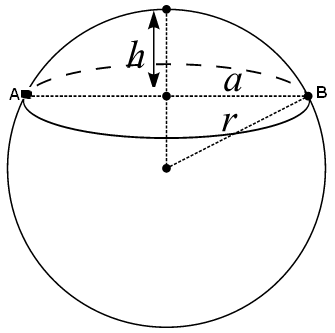## Spherical Cap Calculator

Click on the 2 variables you know:

Sphere Radius 'r' & Cap Height 'h'

Sphere Radius 'r' & Chord 'AB'

Chord 'AB' & Arc 'AB'

Chord 'AB' & Cap Height 'h'

Significant Figures >>>

For easy of calculation, this calculator does all the work for you.

### Formula

1. Volume = 4 * π * r3 / 3

2. Surface Area = 4 * π * r2

3. Volume of Shaded Cap = π * h2 * (3r - h) / 3

4. Surface Area of Shaded cap = 2 * π * r * h

When you know the Sphere Radius 'r' & Cap Height 'h', For example, Sphere Radius = 3, Cap Height 'H' = 1

Then click "calculate" Chord AB = 4.4721, Spherical Cap Radius = 2.2361, Spherical Cap Area = 18.85, Spherical Cap Volume = 8.3776, Arc AB = 5.0464, Sphere Area (Bottom) = 94.248, Total Sphere Area = 113.1, Sphere Volume (Bottom) = 104.72, Total Sphere Volume = 113.1.Here you can make your calculation easier.

Thinkcalculator.com provides you helpful and handy calculator resources.